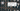# Convert String to Integer - atoi - Leet Code Solution

August 28, 2019## Problem Statement

Implement atoi which converts a string to an integer.

``````Example 1:
Input: "42"
Output: 42

Example 2:
Input: "   -42"
Output: -42

Example 3:
Input: "4193 with words"
Output: 4193

Example 4:
Input: "words and 987"
Output: 0``````

## Algorithm

We need to keep in mind that header includes spaces + and - character only. And, then valid integers. Then, we need to parse integers untill found. If any other character found, it is neglected.

Two special conditions: if the result is out of range (integer overflow, positive), Integer’s max value is returned if number is negative and overflow happened, return max negative value of integer.

• Keep a flag till when we found the first valid integer
• After this encounter, if we got any other character. Just return from there.
• Need to keep the Overflow condition

## Code

``````public class Q8_StringToInteger {
public int myAtoi(String str) {
int s = 0;
boolean neg = false;
boolean foundFirstValidInteger = false;
int l = str.length();

for (int i=0; i<l; i++) {
char c = str.charAt(i);

if (!foundFirstValidInteger) {
if (c == ' ') continue;
if (c == '+') {
foundFirstValidInteger = true;
continue;
}
if (c == '-') {
foundFirstValidInteger = true;
neg = true;
continue;
}
}

if (c >= '0' && c <= '9') {
foundFirstValidInteger = true;
int num = c - '0';

if (s > Integer.MAX_VALUE/10 || (s == Integer.MAX_VALUE/10 && num > 7)) {
if (neg) return Integer.MIN_VALUE;
return Integer.MAX_VALUE;
}
s = s*10 + num;
}
else if (!foundFirstValidInteger) {
return 0;
}
else {
if (neg) return -s;

return s;
}
}

if (neg) return -s;

return s;
}
}``````

## Output

``````"    -42" -> -42
debug 2
"42" -> 42
debug 1
"4193 with words" -> 4193
"words and 987" -> 0
"-91283472332" -> -2147483648
debug 1
"3.14159" -> 3
debug 1
"+-2" -> 0
"  -0012a42" -> -12
"2147483648" -> 2147483647``````